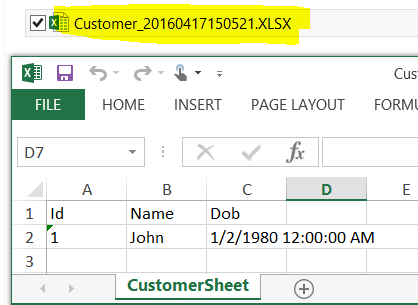### C# - How to Export Stored Procedure Result Set to Excel file dynamically in C#

You are working as C# developer or Dot Net developer and you need to create a program that should execute Stored Procedure from SQL Server database and create an excel file for data returned by Stored Procedure. Let's assume that in in scenario, the Stored Procedure does not accept any parameters.

The Stored Procedure definition can change any time that means that the number of columns returned by Stored Procedure can vary. Our program should be smart enough to create new Excel file without failing when definition of Stored Procedure change.

A new excel file should be generate with Date time on each execution.

Let's create sample table and Stored Procedure first.

```Create table dbo.Customer(
Id INT,
Name VARCHAR(100),
Dob Date)

insert into dbo.Customer values(1,'John','1980-01-02')
go

Create procedure dbo.prc_Customer
AS
BEGIN
Select * From dbo.Customer
END```
```
```
The below program can be used to run Stored Procedure in C# program and write results to Excel file dynamically.

```using System;
using System.Collections.Generic;
using System.Linq;
using System.Text;
using System.IO;
using System.Data;
using System.Data.OleDb;
using System.Data.SqlClient;

namespace TechBrothersIT.com_CSharp_Tutorial
{
class Program
{
static void Main(string[] args)
{
//the datetime and Log folder will be used for error log file in case error occured
string datetime = DateTime.Now.ToString("yyyyMMddHHmmss");
string LogFolder = @"C:\Log\";
try
{
//Declare Variables
//Provide Excel file name that you like to create
string ExcelFileName = "Customer";
//Provide the source folder path where you want to create excel file
string FolderPath = @"C:\Destination\";
//Provide the Stored Procedure Name
string StoredProcedureName = "dbo.prc_Customer";
//Provide Excel Sheet Name
string SheetName = "CustomerSheet";
//Provide the Database in which Stored Procedure exists
string DatabaseName = "TechBrothersIT";
ExcelFileName = ExcelFileName + "_" + datetime;

OleDbConnection Excel_OLE_Con = new OleDbConnection();
OleDbCommand Excel_OLE_Cmd = new OleDbCommand();

//Construct ConnectionString for Excel
string connstring = "Provider=Microsoft.ACE.OLEDB.12.0;" + "Data Source=" + FolderPath + ExcelFileName
+ ";" + "Extended Properties=\"Excel 12.0 Xml;HDR=YES;\"";

//drop Excel file if exists
File.Delete(FolderPath + "\\" + ExcelFileName + ".xlsx");

//Create Connection to SQL Server Database from which you like to export tables to Excel
SqlConnection SQLConnection = new SqlConnection();
SQLConnection.ConnectionString = "Data Source = (local); Initial Catalog =" + DatabaseName + "; " + "Integrated Security=true;";

//Load Data into DataTable from by executing Stored Procedure
string queryString =
"EXEC  " + StoredProcedureName;
DataSet ds = new DataSet();

string TableColumns = "";

// Get the Column List from Data Table so can create Excel Sheet with Header
foreach (DataTable table in ds.Tables)
{
foreach (DataColumn column in table.Columns)
{
TableColumns += column + "],[";
}
}

// Replace most right comma from Columnlist
TableColumns = ("[" + TableColumns.Replace(",", " Text,").TrimEnd(','));
TableColumns = TableColumns.Remove(TableColumns.Length - 2);

//Use OLE DB Connection and Create Excel Sheet
Excel_OLE_Con.ConnectionString = connstring;
Excel_OLE_Con.Open();
Excel_OLE_Cmd.Connection = Excel_OLE_Con;
Excel_OLE_Cmd.CommandText = "Create table " + SheetName + " (" + TableColumns + ")";
Excel_OLE_Cmd.ExecuteNonQuery();

//Write Data to Excel Sheet from DataTable dynamically
foreach (DataTable table in ds.Tables)
{
String sqlCommandInsert = "";
String sqlCommandValue = "";
foreach (DataColumn dataColumn in table.Columns)
{
sqlCommandValue += dataColumn + "],[";
}

sqlCommandValue = "[" + sqlCommandValue.TrimEnd(',');
sqlCommandValue = sqlCommandValue.Remove(sqlCommandValue.Length - 2);
sqlCommandInsert = "INSERT into " + SheetName + "(" + sqlCommandValue + ") VALUES(";

int columnCount = table.Columns.Count;
foreach (DataRow row in table.Rows)
{
string columnvalues = "";
for (int i = 0; i < columnCount; i++)
{
int index = table.Rows.IndexOf(row);
columnvalues += "'" + table.Rows[index].ItemArray[i] + "',";

}
columnvalues = columnvalues.TrimEnd(',');
var command = sqlCommandInsert + columnvalues + ")";
Excel_OLE_Cmd.CommandText = command;
Excel_OLE_Cmd.ExecuteNonQuery();
}

}
Excel_OLE_Con.Close();

}

catch (Exception exception)
{
// Create Log File for Errors
using (StreamWriter sw = File.CreateText(LogFolder
+ "\\" + "ErrorLog_" + datetime + ".log"))
{
sw.WriteLine(exception.ToString());

}

}

}
}
}```

I executed above program and it was able to export the results returned by Stored Procedure to Excel file as shown below.How to Export Stored Procedure Results to Excel File in C#

1.2.3.4.5.6.7.8.9.10.